Name:    2nd Grade Math Proficient Test 12

Multiple Choice
Identify the choice that best completes the statement or answers the question.

1.

Which number sentence will correctly solve this problem?
The children ate 8 slices of a pizza. The pizza was cut into 12 slices.
How many slices were left?
 a. 12 – 8 = 4 d. 20 – 8 = 12 b. 8 + 12 = 20 e. 20 – 4 = 16 c. 8 + 4 = 12

2.

Two brothers can share the money from selling the apples picked from
their grandmother’s apple tree. They pick 100 pounds of apples and sell
them for \$.50 a pound. How much money will each brother get?
 a. \$10.00 d. \$100.00 b. \$25.00 e. \$5.00 c. \$50.00

3.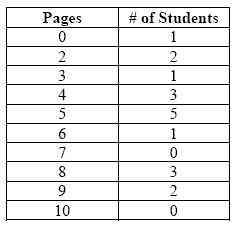The table shows the number of pages students read during silent reading
time. How many students read 5 or more pages?
 a. 17 d. 6 b. 5 e. 10 c. 11

4.

Which ratio represents the number of circles to squares?a. 73 d. 23 b. 37 e. 34 c. 12

5.

Trea has 5 sisters. They have all been asked to help their
Grandmother. Their Grandmother has a list of 12 things she needs the girls to
do. If each girl does her equal share, how many tasks will each girl do?
 a. 1 d. 6 b. 3 e. 2 c. 4

6.

Statistics/Probability and Graphing – Graphing – RIT 181 – 190

Mrs. Smith’s class did a survey to see which was the favorite color of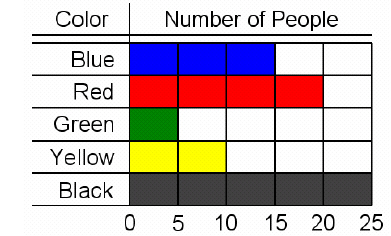Which color was the favorite?
 a. Red d. Blue b. Yellow e. Green c. Black

7.

Statistics/Probability and Graphing – Probability – RIT 181 – 190What is the probability of the spinner stopping on yellow?
 a. possible d. impossible b. likely e. unlikely c. certain

8.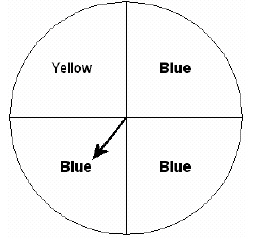What is the probability of the spinner stopping on blue?
 a. 1 to 3 d. 3 to 4 b. 5 to 2 e. 3 to 1 c. 1 to 2

9.

Statistics/Probability and Graphing – Combinations & Permutations – RIT191 – 200
In how many different ways can the letters in the word MATH be
arranged?
 a. 4 d. 16 b. 8 e. 24 c. 12

10.

Statistics/Probability and Graphing – Graphing – RIT 191 – 200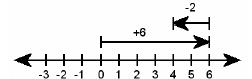What subtraction sentence is modeled on the number line shown above?
 a. 3 + 6 = 9 d. 6 – 4 = 2 b. 6 – 2 = 4 e. 4 – 2 = 2 c. 2 + 6 = 8

11.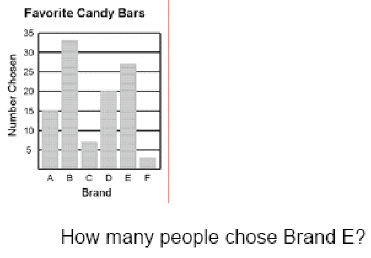a. 7 d. 27 b. 20 e. 33 c. 15

12.

Look at the graph. It represents the number of milkshakes ordered at
“Shakeland” in a three hour period.What is the average number of shakes ordered per hour?
 a. 15 d. 5 b. 10 e. 2 c. 8

13.

The line graph shows the number of students that were absent from a third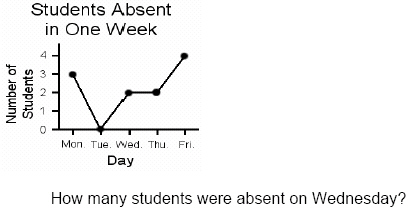a. 0 d. 3 b. 1 e. 4 c. 2

14.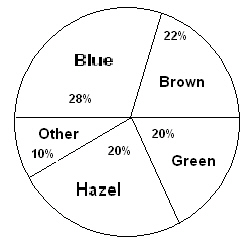What is the most common eye color?
 a. blue d. hazel b. brown e. other c. green

15.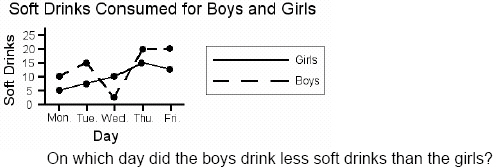a. Monday d. Thursday b. Tuesday e. Friday c. Wednesday

16.

Statistics/Probability and Graphing – Probability and Prediction – RIT 191 –200
Without looking, you pull out only one M&M from a bag that contains 5 red, 10
green, 5 yellow, 5 orange, and 5 blue M&M’s. What color are you most
likely to pull out?
 a. red d. orange b. green e. blue c. yellow

17.

Statistics/Probability and Graphing – Statistics – RIT 191 – 200
In a survey of 30 seventh graders' favorite colors, 5 chose red, 10 chose blue,
7 chose green, and 8 chose yellow. What is the correct tally entry for green?
 a.b.c.d.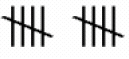e.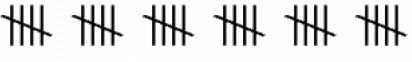18.

Bill received the following scores on 5 tests: 90, 90, 80, 70, 70. What was his
average test score?
 a. 90 d. 75 b. 85 e. 70 c. 80